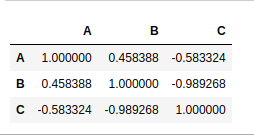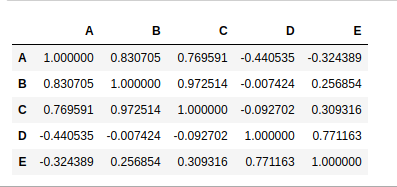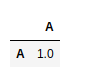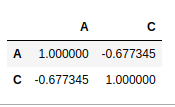# How to Create a Correlation Matrix using Pandas?

• Last Updated : 08 Oct, 2021

Correlation is a statistical technique that shows how two variables are related. Pandas dataframe.corr() method is used for creating the correlation matrix. It is used to find the pairwise correlation of all columns in the dataframe. Any na values are automatically excluded. For any non-numeric data type columns in the dataframe it is ignored.
To create correlation matrix using pandas, these steps should be taken:

1. Obtain the data.
2. Create the DataFrame using Pandas.
3. Create correlation matrix using Pandas

Example 1:

Attention geek! Strengthen your foundations with the Python Programming Foundation Course and learn the basics.

To begin with, your interview preparations Enhance your Data Structures concepts with the Python DS Course. And to begin with your Machine Learning Journey, join the Machine Learning - Basic Level Course

## Python3

 `# import pandas``import` `pandas as pd` `# obtaining the data``data ``=` `{``'A'``: [``45``, ``37``, ``42``],``        ``'B'``: [``38``, ``31``, ``26``],``        ``'C'``: [``10``, ``15``, ``17``]``        ``}``# creation of DataFrame``df ``=` `pd.DataFrame(data)` `# creation of correlation matrix``corrM ``=` `df.corr()` `corrM`

Output:Values at the diagonal shows the correlation of a variable with itself, hence diagonal shows the correlation 1.

Example 2:

## Python3

 `import` `pandas as pd` `data ``=` `{``'A'``: [``45``, ``37``, ``42``, ``50``],``        ``'B'``: [``38``, ``31``, ``26``, ``90``],``        ``'C'``: [``10``, ``15``, ``17``, ``100``],``        ``'D'``: [``60``, ``99``, ``23``, ``56``],``        ``'E'``: [``76``, ``98``, ``78``, ``90``]``        ``}` `df ``=` `pd.DataFrame(data)` `corrM ``=` `df.corr()``corrM`

Output:Example 3:

## Python3

 `import` `pandas as pd` `# Integer and string values can``# never be correlated.``data ``=` `{``'A'``: [``45``, ``37``, ``42``, ``50``],``        ``'B'``: [``'R'``, ``'O'``, ``'M'``, ``'Y'``],``        ``}` `df ``=` `pd.DataFrame(data)` `corrM ``=` `df.corr()``corrM`

Output:Example 4:

## Python3

 `import` `pandas as pd` `data ``=` `{``'A'``: [``45``, ``37``, ``42``, ``50``],``        ``'B'``: [``'R'``, ``'O'``, ``'M'``, ``'Y'``],``        ``'C'``: [``56``, ``67``, ``68``, ``60``],``              ` `        ``}` `df ``=` `pd.DataFrame(data)` `corrM ``=` `df.corr()``corrM`

Output:My Personal Notes arrow_drop_up